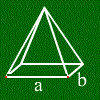# Pyramid calculator

## What is a pyramid?

A pyramid is a solid with a polygon as base area and a point above that base area. Get the pyramid by connecting that point with all the edges of the pyramid.
Often, the term pyramid is just used for pyramids having a square as base area and a point right over the center of that square.
This calculator assumes that this is the fact.

## How to calculate pyramid values?

Formulas depend on what base area you have.
One of the few formulas always right is: The volume equals base area * height /3.
If you want to see some examples, just enter them above.

For further information, move the mouse over one of the words below, and the corresponding part of the pyramid gets marked.side a
diagonal d area
height h side height on a
lateral height lateral area
surface
volume

## Calculating pyramids

Mathepower can calculate pyramids. Just enter base area and height or any other value.